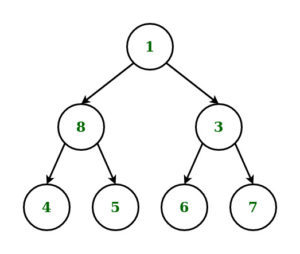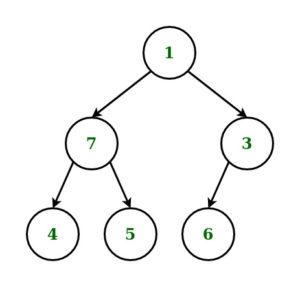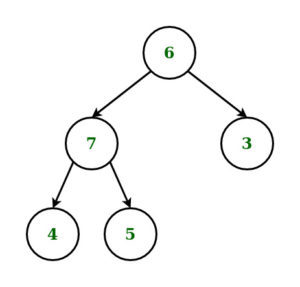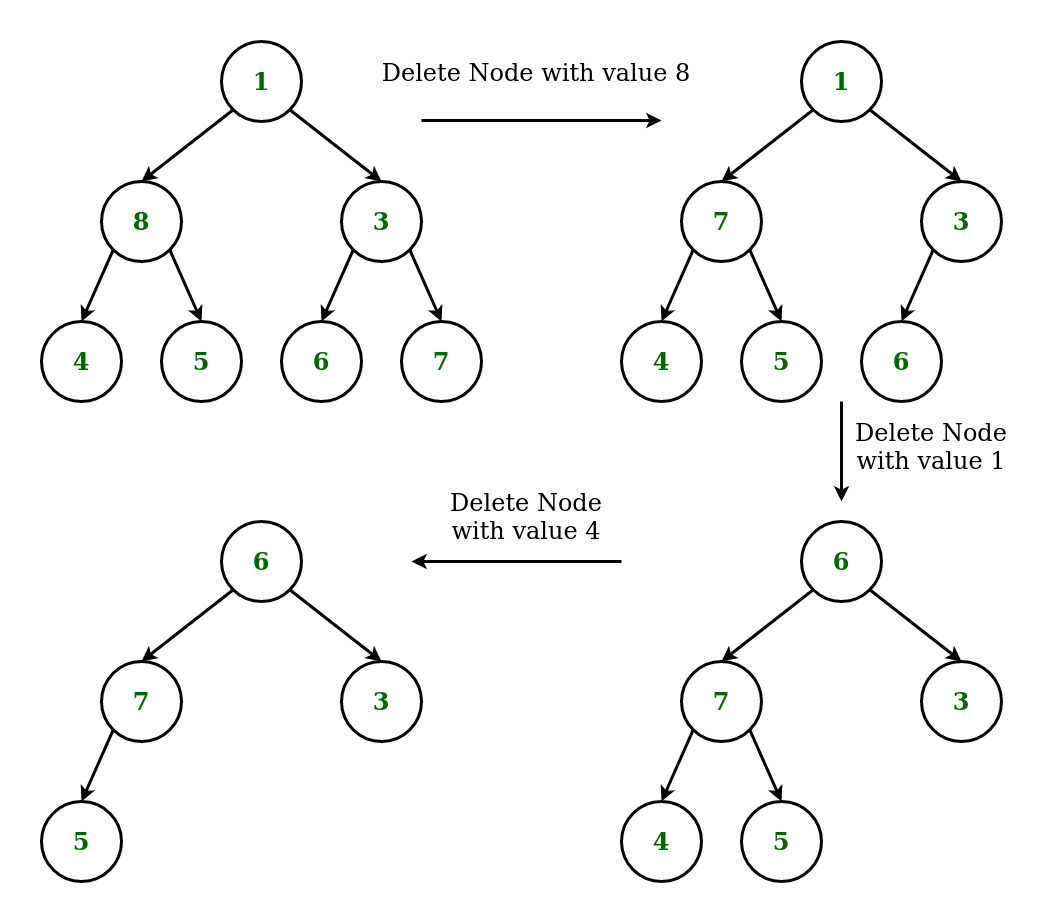Open in App
Not now

# Deletion of a given node K in a Binary Tree using Level Order Traversal

• Difficulty Level : Medium
• Last Updated : 11 May, 2022

Given a binary tree and a node K, the task is to delete the node K from it by making sure that tree shrinks from the bottom (i.e. the deleted node is replaced by bottom-most and rightmost node) using Level Order Traversal.

Examples:

Input: K = 8, Tree =Output:Explanation:

Input: K = 1, Tree =Output:Approach:

• Start searching from the root, the address of node which is to be deleted by traversing in level order-wise.
• Continue Traversing Tree to find the deepest and rightmost node in level order wise to find the deepest and the rightmost node.
• If the node to delete is different from rightmost deepest node, then replace the node to be deleted with rightmost deepest node and delete the later node
• If the node to delete is same as rightmost deepest node, then simply delete the node.

For example: Consider the Example 1 from aboveBelow is the implementation of the above approach.

## C++

 `// C++ implementation to delete a node``// in Binary Tree using Level Order Traversal` `#include ``using` `namespace` `std;` `// Structure of Binary Tree``struct` `node {``    ``int` `data;``    ``node* left;``    ``node* right;``};` `// Function to create new node``// of a Binary Tree.``node* newnode(``int` `d)``{``    ``node* temp = ``new` `node;``    ``temp->data = d;``    ``temp->left = temp->right = NULL;``    ``return` `temp;``}` `// Function for Level Order``// Traversal of Binary Tree``void` `levelorder(node* curr)``{` `    ``// Queue to store the nodes``    ``// in a level``    ``queue q;``    ``if` `(curr)``        ``q.push(curr);` `    ``// Loop to Traverse the Binary``    ``// Tree in Level Order``    ``while` `(!q.empty()) {``        ``curr = q.front();``        ``q.pop();``        ``cout << curr->data << ``" "``;``        ``if` `(curr->left)``            ``q.push(curr->left);``        ``if` `(curr->right)``            ``q.push(curr->right);``    ``}``}` `// Function to Delete the deepest``// right-most node of the Binary Tree``void` `deletedeepest(node* root,``                ``node* temp)``{``    ``queue q;``    ``q.push(root);` `    ``// Loop to Traverse Binary Tree``    ``// in level order and delete Node``    ``while` `(!q.empty()) {``        ``node* T = q.front();``        ``q.pop();``        ``if` `(T->right == temp) {``            ``T->right = NULL;``            ``delete` `(temp);``            ``return``;``        ``}``        ``else``            ``q.push(T->right);``        ``if` `(T->left == temp) {``            ``T->left = NULL;``            ``delete` `(temp);``            ``return``;``        ``}``        ``else``            ``q.push(T->left);``    ``}``}` `// Function to Delete Node``// in Binary Tree``node* deletenode(node* root, ``int` `data)``{``    ``if` `(root == NULL)``        ``return` `NULL;` `    ``// Condition if the Root node``    ``// is a leaf node.``    ``if` `(root->left == NULL``        ``&& root->right == NULL) {``        ``if` `(root->data == data) {``            ``return` `NULL;``        ``}``        ``else``            ``return` `root;``    ``}``    ``queue q;``    ``q.push(root);``    ``node* temp = NULL;``    ``node* nodetodelete = NULL;` `    ``// Loop to Traverse Tree in``    ``// Level Order and find the``    ``// Rightmost Deepest Node of the``    ``// Binary Tree and Node to be Deleted``    ``while` `(!q.empty()) {``        ``temp = q.front();``        ``q.pop();``        ``if` `(temp->data == data) {``            ``nodetodelete = temp;``        ``}``        ``if` `(temp->left) {``            ``q.push(temp->left);``        ``}``        ``if` `(temp->right) {``            ``q.push(temp->right);``        ``}``    ``}` `    ``// if node to be deleted is not found``    ``if` `(nodetodelete != NULL) {` `        ``// Condition to check if node to delete``        ``// is not same as node to replace``        ``if` `(temp != nodetodelete) {``            ``int` `t = temp->data;``            ``deletedeepest(root, temp);``            ``nodetodelete->data = t;``        ``}` `        ``// if node to delete is also``        ``// rightmost deepest node``        ``else` `{``            ``deletedeepest(root, temp);``        ``}``    ``}``    ``return` `root;``}` `// Driver Code``int` `main()``{` `    ``// Construction of Tree``    ``node* root = newnode(1);``    ``root->left = newnode(8);``    ``root->right = newnode(3);``    ``root->left->left = newnode(4);``    ``root->left->right = newnode(5);``    ``root->right->left = newnode(6);``    ``root->right->right = newnode(7);` `    ``cout << ``"Original Tree: "``;``    ``levelorder(root);` `    ``// Deleting node with key 8``    ``root = deletenode(root, 8);``    ``cout << endl;``    ``cout << ``"Deleting node with key 8: "``;``    ``levelorder(root);` `    ``// Deleting node with key 1``    ``root = deletenode(root, 1);``    ``cout << endl;``    ``cout << ``"Deleting node with key 1: "``;``    ``levelorder(root);` `    ``// Deleting node with key 4``    ``root = deletenode(root, 4);``    ``cout << endl;``    ``cout << ``"Deleting node with key 4: "``;``    ``levelorder(root);``}`

## Java

 `// Java implementation to delete a node``// in Binary Tree using Level Order Traversal``import` `java.util.LinkedList;``import` `java.util.Queue;` `// Binary Tree``class` `TreeNode``{``    ``int` `data;``    ``TreeNode left = ``null``;``    ``TreeNode right = ``null``;``    ` `    ``TreeNode(``int` `data)``    ``{``        ``this``.data = data;``    ``}``}` `class` `BinaryTreeDeleteKNode{` `public` `TreeNode insert(TreeNode root,``                    ``int` `value)``{``    ``if` `(root == ``null``)``    ``{``        ``root = ``new` `TreeNode(value);``        ``return` `root;``    ``}``    ` `    ``// Do level order traversal and add``    ``// node to first null``    ``Queue q = ``new` `LinkedList();``    ``q.add(root);``    ` `    ``while` `(!q.isEmpty())``    ``{``        ``TreeNode tn = q.remove();``        ``if` `(tn.left != ``null``)``        ``{``            ``q.add(tn.left);``        ``}``        ``else``        ``{``            ``tn.left = ``new` `TreeNode(value);``            ``return` `root;``        ``}``        ``if` `(tn.right != ``null``)``        ``{``            ``q.add(tn.right);``        ``}``        ``else``        ``{``            ``tn.right = ``new` `TreeNode(value);``            ``return` `root;``        ``}``    ``}``    ``return` `root;``}` `// Function for Level Order``// Traversal of Binary Tree``public` `void` `levelOrder(TreeNode root)``{``    ``if` `(root == ``null``)``    ``{``        ``System.out.println(``"Tree is empty!!"``);``    ``}``    ` `    ``// Queue to store the nodes``    ``// in a level``    ``Queue q = ``new` `LinkedList();``    ``q.add(root);``    ` `    ``// Loop to Traverse the Binary``    ``// Tree in Level Order``    ``while` `(!q.isEmpty())``    ``{``        ``TreeNode tn = q.remove();``        ``System.out.print(tn.data + ``" "``);``        ` `        ``if` `(tn.left != ``null``)``            ``q.add(tn.left);``        ``if` `(tn.right != ``null``)``            ``q.add(tn.right);``    ``}``}` `// Function to delete node node with value K``public` `TreeNode deleteIn(TreeNode root, ``int` `k)``{``    ``if` `(root == ``null``)``        ``return` `root;``    ` `    ``// Do level order traversal if node found``    ``// with the value k then select that node``    ``// keep traversal till we find deepest``    ``// node with empty value keep track of``    ``// the parent node of the deepest node``    ``TreeNode searchedNode = ``null``;``    ``TreeNode tn = ``null``;``    ` `    ``Queue q = ``new` `LinkedList();``    ``TreeNode deepestNodeParent = ``null``;``    ``q.add(root);``    ` `    ``while` `(!q.isEmpty())``    ``{``        ``boolean` `isParent = ``false``;``        ``tn = q.remove();``        ` `        ``if` `(searchedNode == ``null` `&& tn.data == k)``        ``{``            ``searchedNode = tn;``        ``}``        ``if` `(tn.left != ``null``)``        ``{``            ``q.add(tn.left);``            ``isParent = ``true``;``        ``}``        ``if` `(tn.right != ``null``)``        ``{``            ``q.add(tn.right);``            ``isParent = ``true``;``        ``}``        ``if` `(isParent) deepestNodeParent = tn;``    ``}` `    ``if` `(searchedNode == ``null``)``    ``{``        ``System.out.print(``"Node with value '"` `+ k +``                        ``"' not exists."``);``        ``return` `root;``    ``}``    ``searchedNode.data = tn.data;``    ``if` `(deepestNodeParent != ``null` `&&``        ``deepestNodeParent.left != ``null` `&&``        ``deepestNodeParent.left.data == tn.data)``    ``{``    ``deepestNodeParent.left = ``null``;``    ``}``    ``else``    ``{``        ``deepestNodeParent.right = ``null``;``    ``}``    ``return` `root;``}` `// Driver code``public` `static` `void` `main(String[] args)``{``    ``TreeNode node = ``null``;``    ``BinaryTreeDeleteKNode binaryTreeDeleteKNode = ``new` `BinaryTreeDeleteKNode();``    ` `    ``// Construction of Tree``    ``node = binaryTreeDeleteKNode.insert(node, ``1``);``    ``node = binaryTreeDeleteKNode.insert(node, ``8``);``    ``node = binaryTreeDeleteKNode.insert(node, ``3``);``    ``node = binaryTreeDeleteKNode.insert(node, ``4``);``    ``node = binaryTreeDeleteKNode.insert(node, ``5``);``    ``node = binaryTreeDeleteKNode.insert(node, ``6``);``    ``node = binaryTreeDeleteKNode.insert(node, ``7``);``    ` `    ``System.out.print(``"Original Tree: "``);``    ``binaryTreeDeleteKNode.levelOrder(node);``    ` `    ``// Deleting node with key 8``    ``node = binaryTreeDeleteKNode.deleteIn(node,``8``);``    ``System.out.print(``"\nDeleting node with key 8: "``);``    ``binaryTreeDeleteKNode.levelOrder(node);``    ` `    ``// Deleting node with key 1``    ``node = binaryTreeDeleteKNode.deleteIn(node,``1``);``    ``System.out.print(``"\nDeleting node with key 1: "``);``    ``binaryTreeDeleteKNode.levelOrder(node);``    ` `    ``// Deleting node with key 4``    ``node = binaryTreeDeleteKNode.deleteIn(node,``4``);``    ``System.out.print(``"\nDeleting node with key 4: "``);``    ``binaryTreeDeleteKNode.levelOrder(node);``}``}` `// This code is contributed by anshulgtbit91`

## Python3

 `# Python3 implementation to delete a node``# in Binary Tree using Level Order Traversal``from` `collections ``import` `deque` `# Structure of Binary Tree``class` `Node:``    ` `    ``def` `__init__(``self``, x):``        ` `        ``self``.data ``=` `x``        ``self``.left ``=` `None``        ``self``.right ``=` `None` `# Function for Level Order``# Traversal of Binary Tree``def` `levelorder(curr):``    ` `    ``# Queue to store the nodes``    ``# in a level``    ``q ``=` `deque()` `    ``if` `(curr):``        ``q.append(curr)` `    ``# Loop to Traverse the Binary``    ``# Tree in Level Order``    ``while` `len``(q) > ``0``:``        ``curr ``=` `q.popleft()``        ``#q.pop()``        ``print``(curr.data, end ``=` `" "``)``        ` `        ``if` `(curr.left):``            ``q.append(curr.left)``        ``if` `(curr.right):``            ``q.append(curr.right)` `# Function to Delete the deepest``# right-most node of the Binary Tree``def` `deletedeepest(root, temp):``    ` `    ``q ``=` `deque()``    ``q.append(root)` `    ``# Loop to Traverse Binary Tree``    ``# in level order and delete Node``    ``while` `(``len``(q) > ``0``):``        ``T ``=` `q.popleft()``        ``#q.pop()``        ` `        ``if` `(T.right ``=``=` `temp):``            ``T.right ``=` `None``            ``#delete (temp)``            ``return``        ``else``:``            ``q.append(T.right)``            ` `        ``if` `(T.left ``=``=` `temp):``            ``T.left ``=` `None``            ``#delete (temp)``            ``return``        ``else``:``            ``q.append(T.left)` `# Function to Delete Node``# in Binary Tree``def` `deletenode(root, data):``    ` `    ``if` `(root ``=``=` `None``):``        ``return` `None` `    ``# Condition if the Root node``    ``# is a leaf node.``    ``if` `(root.left ``=``=` `None` `and``        ``root.right ``=``=` `None``):``        ``if` `(root.data ``=``=` `data):``            ``return` `None``        ``else``:``            ``return` `root` `    ``q ``=` `deque()``    ``q.append(root)``    ``temp ``=` `None``    ``nodetodelete ``=` `None` `    ``# Loop to Traverse Tree in``    ``# Level Order and find the``    ``# Rightmost Deepest Node of the``    ``# Binary Tree and Node to be Deleted``    ``while` `(``len``(q) > ``0``):``        ``temp ``=` `q.popleft()``        ``#q.pop()``        ` `        ``if` `(temp.data ``=``=` `data):``            ``nodetodelete ``=` `temp``        ``if` `(temp.left):``            ``q.append(temp.left)``        ``if` `(temp.right):``            ``q.append(temp.right)` `    ``# If node to be deleted is not found``    ``if` `(nodetodelete !``=` `None``):``        ` `        ``# Condition to check if node to delete``        ``# is not same as node to replace``        ``if` `(temp !``=` `nodetodelete):``            ``t ``=` `temp.data``            ``deletedeepest(root, temp)``            ``nodetodelete.data ``=` `t``            ` `        ``# If node to delete is also``        ``# rightmost deepest node``        ``else``:``            ``deletedeepest(root, temp)``            ` `    ``return` `root` `# Driver Code``if` `__name__ ``=``=` `'__main__'``:``    ` `    ``# Construction of Tree``    ``root ``=` `Node(``1``)``    ``root.left ``=` `Node(``8``)``    ``root.right ``=` `Node(``3``)``    ``root.left.left ``=` `Node(``4``)``    ``root.left.right ``=` `Node(``5``)``    ``root.right.left ``=` `Node(``6``)``    ``root.right.right ``=` `Node(``7``)` `    ``print``(``"Original Tree: "``, end ``=` `"")``    ``levelorder(root)` `    ``# Deleting node with key 8``    ``root ``=` `deletenode(root, ``8``)``    ``print``()``    ``print``(``"Deleting node with key 8: "``, end ``=` `"")``    ``levelorder(root)` `    ``# Deleting node with key 1``    ``root ``=` `deletenode(root, ``1``)``    ``print``()``    ``print``(``"Deleting node with key 1: "``, end ``=` `"")``    ``levelorder(root)` `    ``# Deleting node with key 4``    ``root ``=` `deletenode(root, ``4``)``    ``print``()``    ``print``(``"Deleting node with key 4: "``, end ``=` `"")``    ``levelorder(root)``    ` `# This code is contributed by mohit kumar 29`

## C#

 `// C# implementation to delete a node``// in Binary Tree using Level Order Traversal` `using` `System;``using` `System.Collections.Generic;`  `// Binary Tree``public` `class` `TreeNode``{``    ``public` `int` `data;``    ``public` `TreeNode left = ``null``;``    ``public` `TreeNode right = ``null``;``    ` `    ``public` `TreeNode(``int` `data)``    ``{``        ``this``.data = data;``    ``}``}` `public` `class` `BinaryTreeDeleteKNode{` `public` `TreeNode insert(TreeNode root,``                    ``int` `value)``{``    ``if` `(root == ``null``)``    ``{``        ``root = ``new` `TreeNode(value);``        ``return` `root;``    ``}``    ` `    ``// Do level order traversal and add``    ``// node to first null``    ``Queue q = ``new` `Queue();``    ``q.Enqueue(root);``    ` `    ``while` `(q.Count!=0)``    ``{``        ``TreeNode tn = q.Dequeue();``        ``if` `(tn.left != ``null``)``        ``{``            ``q.Enqueue(tn.left);``        ``}``        ``else``        ``{``            ``tn.left = ``new` `TreeNode(value);``            ``return` `root;``        ``}``        ``if` `(tn.right != ``null``)``        ``{``            ``q.Enqueue(tn.right);``        ``}``        ``else``        ``{``            ``tn.right = ``new` `TreeNode(value);``            ``return` `root;``        ``}``    ``}``    ``return` `root;``}` `// Function for Level Order``// Traversal of Binary Tree``public` `void` `levelOrder(TreeNode root)``{``    ``if` `(root == ``null``)``    ``{``        ``Console.WriteLine(``"Tree is empty!!"``);``    ``}``    ` `    ``// Queue to store the nodes``    ``// in a level``    ``Queue q = ``new` `Queue();``    ``q.Enqueue(root);``    ` `    ``// Loop to Traverse the Binary``    ``// Tree in Level Order``    ``while` `(q.Count!=0)``    ``{``        ``TreeNode tn = q.Dequeue();``        ``Console.Write(tn.data + ``" "``);``        ` `        ``if` `(tn.left != ``null``)``            ``q.Enqueue(tn.left);``        ``if` `(tn.right != ``null``)``            ``q.Enqueue(tn.right);``    ``}``}` `// Function to delete node node with value K``public` `TreeNode deleteIn(TreeNode root, ``int` `k)``{``    ``if` `(root == ``null``)``        ``return` `root;``    ` `    ``// Do level order traversal if node found``    ``// with the value k then select that node``    ``// keep traversal till we find deepest``    ``// node with empty value keep track of``    ``// the parent node of the deepest node``    ``TreeNode searchedNode = ``null``;``    ``TreeNode tn = ``null``;``    ` `    ``Queue q = ``new` `Queue();``    ``TreeNode deepestNodeParent = ``null``;``    ``q.Enqueue(root);``    ` `    ``while` `(q.Count!=0)``    ``{``        ``bool` `isParent = ``false``;``        ``tn = q.Dequeue();``        ` `        ``if` `(searchedNode == ``null` `&& tn.data == k)``        ``{``            ``searchedNode = tn;``        ``}``        ``if` `(tn.left != ``null``)``        ``{``            ``q.Enqueue(tn.left);``            ``isParent = ``true``;``        ``}``        ``if` `(tn.right != ``null``)``        ``{``            ``q.Enqueue(tn.right);``            ``isParent = ``true``;``        ``}``        ``if` `(isParent) deepestNodeParent = tn;``    ``}` `    ``if` `(searchedNode == ``null``)``    ``{``        ``Console.Write(``"Node with value '"` `+ k +``                        ``"' not exists."``);``        ``return` `root;``    ``}``    ``searchedNode.data = tn.data;``    ``if` `(deepestNodeParent != ``null` `&&``        ``deepestNodeParent.left != ``null` `&&``        ``deepestNodeParent.left.data == tn.data)``    ``{``    ``deepestNodeParent.left = ``null``;``    ``}``    ``else``    ``{``        ``deepestNodeParent.right = ``null``;``    ``}``    ``return` `root;``}``// Driver Code``public` `static` `void` `Main()``{``    ``TreeNode node = ``null``;``    ``BinaryTreeDeleteKNode binaryTreeDeleteKNode = ``new` `BinaryTreeDeleteKNode();``    ` `    ``// Construction of Tree``    ``node = binaryTreeDeleteKNode.insert(node, 1);``    ``node = binaryTreeDeleteKNode.insert(node, 8);``    ``node = binaryTreeDeleteKNode.insert(node, 3);``    ``node = binaryTreeDeleteKNode.insert(node, 4);``    ``node = binaryTreeDeleteKNode.insert(node, 5);``    ``node = binaryTreeDeleteKNode.insert(node, 6);``    ``node = binaryTreeDeleteKNode.insert(node, 7);``    ` `    ``Console.Write(``"Original Tree: "``);``    ``binaryTreeDeleteKNode.levelOrder(node);``    ` `    ``// Deleting node with key 8``    ``node = binaryTreeDeleteKNode.deleteIn(node,8);``    ``Console.Write(``"\nDeleting node with key 8: "``);``    ``binaryTreeDeleteKNode.levelOrder(node);``    ` `    ``// Deleting node with key 1``    ``node = binaryTreeDeleteKNode.deleteIn(node,1);``    ``Console.Write(``"\nDeleting node with key 1: "``);``    ``binaryTreeDeleteKNode.levelOrder(node);``    ` `    ``// Deleting node with key 4``    ``node = binaryTreeDeleteKNode.deleteIn(node,4);``    ``Console.Write(``"\nDeleting node with key 4: "``);``    ``binaryTreeDeleteKNode.levelOrder(node);` `    ``}``}` `// This code is contributed by jana_sayantan.`

## Javascript

 ``

Output:

```Original Tree: 1 8 3 4 5 6 7
Deleting node with key 8: 1 7 3 4 5 6
Deleting node with key 1: 6 7 3 4 5
Deleting node with key 4: 6 7 3 5```

My Personal Notes arrow_drop_up